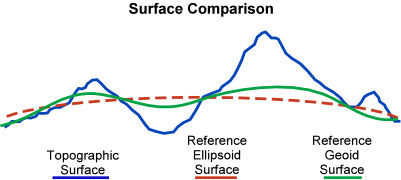# Coordinates of Metsähovi

The location of a radio observatory needs to be known precisely. The Metsähovi 14-metre radio telescope's coordinates are one of the most accurately known in Finland. In fact, the Finnish coordinates are partly based on measurement done in Metsähovi.

## Coordinates of Metsähovi

Nowadays there are four different main geodetic techniques that are used to compare accurate coordinates; the Global Positioning System (GPS), Very Long Baseline Interferometry (VLBI), Satellite Laser Ranging (SLR) and Doppler Orbitography and Radiopositioning Integrated by Satellite (DORIS).

Since the tracking network equipped with the instruments of those four techniques is evolving and the period of data available increases with time, the International Terrestial Reference Frame (ITRF) is constantly being updated. 11 realizations were set up from 1988. The latest is the ITRF2005 (the ITRF web page).

## Geodetic coordinates

To be able to use a coordinate system based on using the ellipsoid for example to report locations of points on the surface of the Earth, we must have a third coordinate besides the latitude and longitude. The height from the ellipsoid is usually marked with h. Latitude, longitude and h are called the geodetic coordinates. The coordinates of a point on the surface of the Earth on the ellipsoid stand for the coordinates of a point projected to a surface of an ellipsoid. Geodetic height h differs from the orthometric height H (see the explanation below, also called the topographic height) with the height of the geoid N (see the explanation below), h = H + N.

 Topographic Height H = Ellipsoidal Height h - Geoid Height NFigure 1. The topographic surface of the Earth H, the reference ellipsoid h and the reference geoid surface N.

### Conventional Terrestial System

Conventional Terrestial System, CTS, is geosentric and the axis are marked with X, Y and Z. X coordinates are positive in 'the Atlantic side' and negative in 'the Pacific side'. Z coordinates are positive in the northern hemisphere and negative in the southern. Y coordinates are positive in the eastern side of the Greenwich meridian and negative in the western side. The CTS is calculated from the ITRF.

### The starting values

The coordinates below are given in the ITRF2005 coordinate system. The ITRF2005 coordinates of the GPS antenna of Metsähovi are used as starting values.

The starting values are:

Lat.: 60o 13' 2.89765''
Long.: 24o 23' 43.14673''
Height h: 94.593 m

X: 2892570.871
Y: 1311843.375
Z: 5512634.085

We must add to these a measured vector of:

dX: 13.854
dY: -128.114
dZ: 5.314

### The ITRF2005 coordinates of Metsähovi

From the values above we calculate the coordinates of Metsähovi:

Lat.: 60o 13' 4.11295''
Long.: 24o 23' 35.19791''
Height h: 79.191 m

X: 2892584.725
Y: 1311715.261
Z: 5512639.399

Above, the height value is the ellipsoid height. To calculate the orthometric height (height above the sea level) we must add the FIN2000 geoid model (see explanation) and we get the height in the N60 system (see explanation):

H: 60.28 m

The accuracy of the H value is a couple of centimeters.

### Some explanations

• Geoid: If all the water in the oceans was of homogenic material and there were no other forces than gravity interacting with it, the sea level would place oneself to an equilibrium of gravity's equipotential, which is called a geoid. The geoid is calculated nowadays gravimetricly.
• Ellipsoidal height h: The geoid height N is given in comparison to the ellipsoid (nowadays the Geodetic Reference System 80, GRS80). The GRS80 is geosentric. N is more than 0, when the geoid is above the ellipsoid and, N is less than 0, when the geoid is lower than the ellipsoid.
• Orthometric height H: is the distance of a point from the geoid surface along the vertical line. Can also be called topographic height.
• FIN2000: To calculate the geoid height H we need a geoid model. FIN2000 is a geoid model which applies in the conditions of Finland.
• N60: The most precise way to measure heights is to use (precise) levelling. In the nation wide height system N60 the datum level is the average sea level of Helsinki in the year 1960.
This service is provided by: